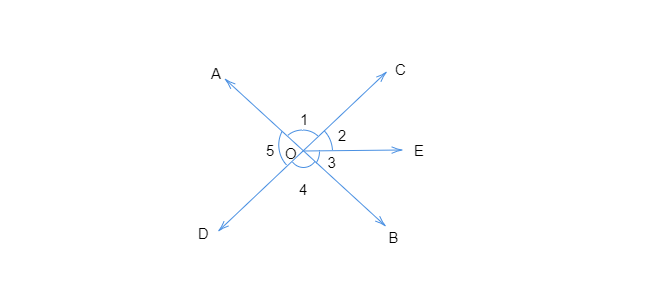# In the adjoining figure:$(i)$ Is $\angle 1$ adjacent to $\angle 2$?$(ii)$ Is $\angle AOC$ adjacent to $\angle AOE$?$(iii)$ Do $\angle COE$ and $\angle EOD$ form a linear pair?$(iv)$ Are $\angle BOD$ and $\angle DOA$ supplementary?$(v)$ Is $\angle 1$ vertically opposite to $\angle 4$?$(vi)$ What is the vertically opposite angle of $\angle 5$?"

To do:

We have to answer the given questions by observing the given figure.

Solution:

(i) Yes, $\angle 1$ and $\angle 2$ are adjacent angles.

$\angle 1$ and $\angle 2$ have a common vertex $O$, a common arm $OC$ and $OA$ and $OE$ are on both sides of the common arm $OC$.

(ii) No, $\angle AOC$ is not adjacent to $\angle AOE$

$OC$ and $OE$ do not lie on either side of the common arm $OA$.

(iii) Yes, $\angle COE$ and $\angle EOD$ form a linear pair.

$CO$ and $OD$ form a straight line and the sum of $\angle COE$ and $\angle EOD$ is $180^o$

(iv) Yes, $\angle BOD$ and $\angle DOA$ are supplementary.

$\angle BOD$ and $\angle DOA$ have a common vertex $O$, a common arm $OD$ and $OA$ and $OB$ are on both sides of the common arm $OD$.

(v) Yes, $\angle 1$ is vertically opposite to $\angle 4$.

(vi) Vertically opposite angle of $\angle 5$ is $\angle BOC$.